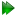Subsections

frame_order.permute_axesSynopsis

Permute the axes of the motional eigenframe to switch between local minima.

Defaults

frame_order.permute_axes(permutation=`A')

Keyword arguments

permutation: Which of the two permutations `A' or `B' to create. Three permutations are possible, and `A' and `B' select those which are not the starting combination.

Description

The isotropic and pseudo-elliptic cone frame order models consist of multiple solutions as the optimisation space contains multiple local minima. Because of the constraint cone_theta_x cone_theta_y in the pseudo-ellipse model, there are exactly three local minima (out of 6 possible permutations). However the cone_theta_x == cone_theta_y condition of the isotropic cone collapses this to two minima. The multiple minima correspond to permutations of the motional system - the eigenframe x, y and z-axes as well as the cone opening angles cone_theta_x, cone_theta_y, and cone_sigma_max associated with these axes. But as the mechanics of the cone angles is not identical to that of the torsion angle, only one of the three local minima is the global minimum.

When optimising the pseudo-elliptic models, specifically the `pseudo-ellipse' and `pseudo-ellipse, torsionless' model, any of the three local minima can be found. Convergence to the global minimum is not guaranteed. Therefore this user function can be used to permute the motional system to jump from one local minimum to the other. Optimisation will be required as the permuted parameters will not be exactly at the minimum.

Please see Table 17.7 on page.

 Condition Permutation name Cone angles Axes x < y < z Self [x, y, z] [x, y, z] A [x, z, y] [-z, y, x] B [y, z, x] [z, x, y] x < z < y Self [x, y, z] [x, y, z] A [x, z, y] [-z, y, x] B [z, y, x] [x, -z, y] z < x < y Self [x, y, z] [x, y, z] A [z, x, y] [y, z, x] B [z, y, x] [x, -z, y]

In this table, the condition and cone angle values [x, y, z] correspond to cone_theta_x, cone_theta_y, and cone_sigma_max.

Prompt examples

For combination `A', simply type:

[numbers=none]
relax> frame_order.permute_axes('A')

The relax user manual (PDF), created 2020-08-26.